# Advanced Tutorial Lesson 5: Analyzing a Balanced BJT Mixer

 Tutorial Project: Analyzing a Balanced BJT Mixer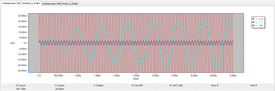Objective: In this project, you will build and analyze a balanced mixer circuit and examine its spectral performance. Concepts/Features: Bipolar Junction Transistor Balanced Mixer Operating Point Analysis Transient Test Fourier Analysis Fundamental Frequency Spectral Contents Minimum Version Required: All versions

## What You Will Learn

In this tutorial you will build and test a balanced mixer circuit using differential BJT pairs. You will perform Fourier analysis of your mixer circuit to examine the various spectral contents present at the IF output of the mixer.

## Putting the Mixer Circuit Together

The following is a list of parts needed for this part of the tutorial lesson:

Part Name Part Type Part Value
VRF Voltage Source Waveform TBD
VLO Voltage Source Waveform TBD
VCC Voltage Source DC, 10
VBIAS Voltage Source DC, -2
IEE Current Source DC, 300u
RC1 - RC2 Resistor 5k
C1 Capacitor 100p
L1 Inductor 100u
K1 - K3 Ideal Transformer n = 1
Q1 - Q6 Generic NPN BJT Defaults (beta = 100)
RF, LO, IF Voltage Probe Marker N/A

The figure below shows the balanced mixer: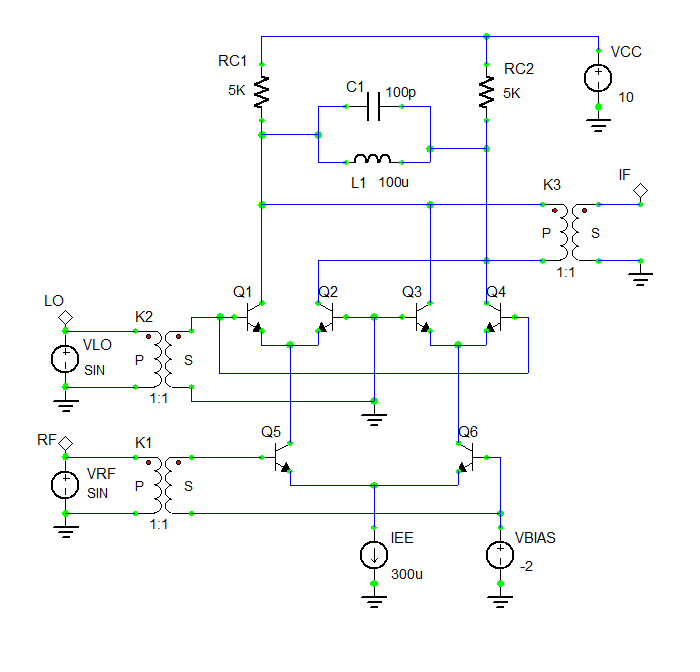The schematic of the balanced BJT mixer.

Set the waveforms of the two voltage sources VRF and VLO according to the following table:

 Offset Voltage 0 2m 14.25Meg 0 0

 Offset Voltage 0 90m 12.75Meg 0 0

Place all the parts in the Schematic Editor and connect them as shown above. The three 1:1 transformers K1, K2 and K3 at the two inputs (RF and LO) and at the output (IF) have been used as buffers for isolation. The output transformer K3 provides a convenient way to get the differential output at the collectors of Q1 and Q2.

## Running an Operating Point Analysis

Run a DC Bias Test of your mixer circuit to verify the operating point performance of all the six BJT transistors. Make sure to check the Table checkbox in the Test Panel before running the test. Otherwise, no output data will be generated. The table below shows the test results: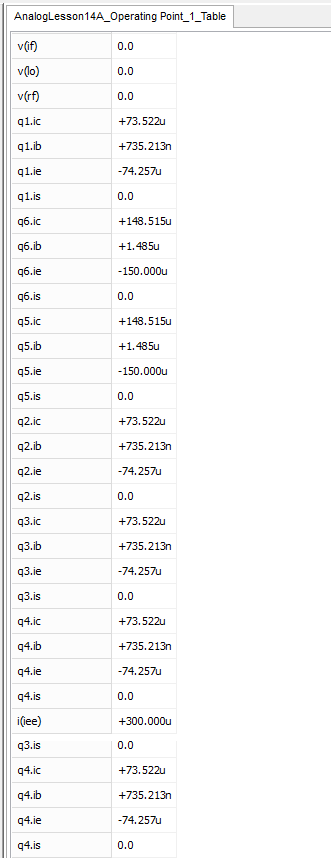The tabulated results of DC bias test.

## Running a Transient Test of the Mixer Circuit

Run a Transient Test of this circuit with the parameters specified below:

 Start Time 0 5u 1n 1n v(rf), v(lo), v(if)

The results for the RF, LO and IF voltages are shown in the figure below:The graph of RF, LO and IF voltages in the mixer circuit.

You can zoom to a selected plot to better examine its details. First, activate the graph window. Then, click the "Zoom to Selected Plot"button of the Graph Toolbar and click on the IF plot to select it. You can see that frequency of the IF signal is fIF = fRF - fLO = 14.25MHz - 12.75MHz = 1.5MHz.

## Performing a Spectral Analysis of the Mixer Circuit

In order to find out how pure the IF signal is, perform a Fourier analysis of the output voltage of your mixer circuit. As you did in the Analog Tutorial Lesson 13, this can be done from the Transient Test Panel. In the "Fourier Transform Settings dialog", set the positive node to 13 and the reference node to 0 (ground). Run six transient tests, each with a different fundamental frequency as listed below:

• fo = fIF = 1.5MHz
• fo = fRF = 14.25MHz
• fo = fLO = 12.75MHz
• fo = fRF + fLO = 27MHz
• fo = 2*fRF - fLO = 15.75MHz
• fo = 2*fLO - fRF = 11.25MHz

The six Fourier content bar charts are shown in the figures below. As you can see from these figures, there are no harmonics of the IF frequency present at the output. The spectral contents at the RF and LO as well as the sum and image frequencies are all about 10 times smaller than that of the IF frequency.The spectral contents of the IF voltage at the IF frequency fo = 1.5MHz and its harmonics.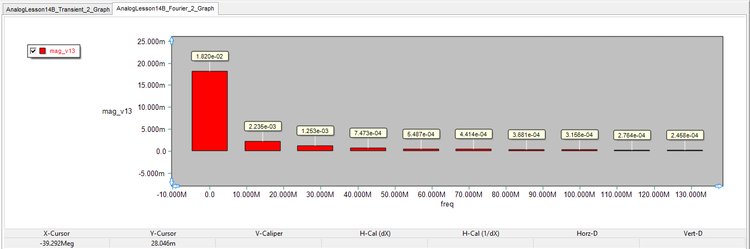The spectral contents of the IF voltage at the RF frequency fo = 14.25MHz and its harmonics.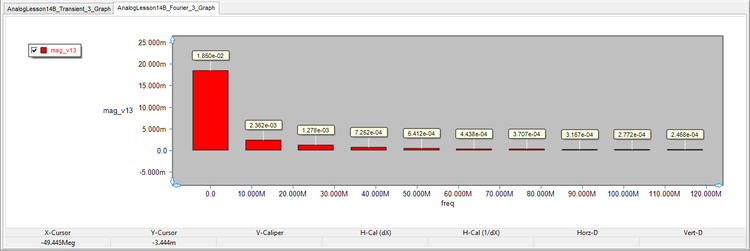The spectral contents of the IF voltage at the LO frequency fo = 12.75MHz and its harmonics.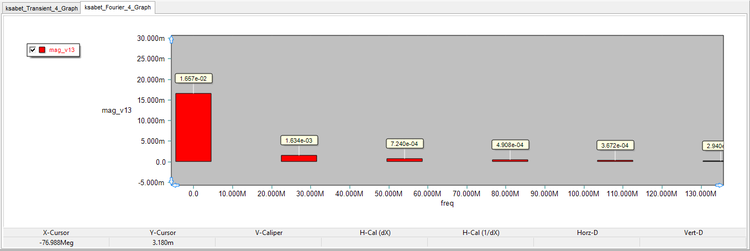The spectral contents of the IF voltage at the sum frequency fo = 27MHz and its harmonics.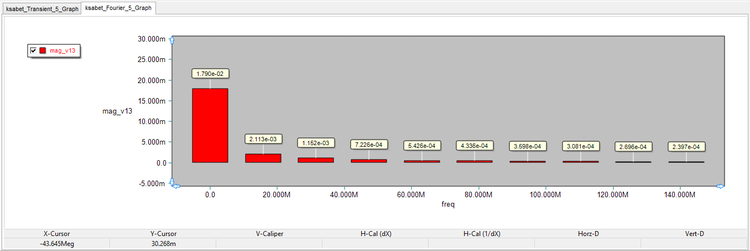The spectral contents of the IF voltage at the image frequency fo = 15.75MHz and its harmonics.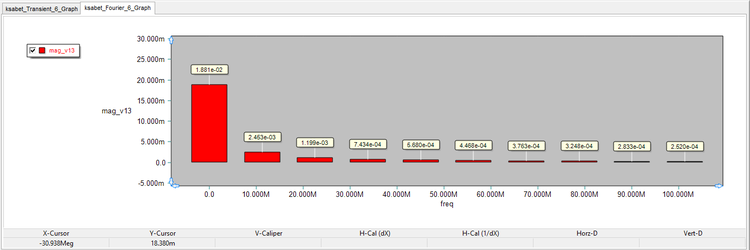The spectral contents of the IF voltage at the image frequency fo = 11.25MHz and its harmonics.